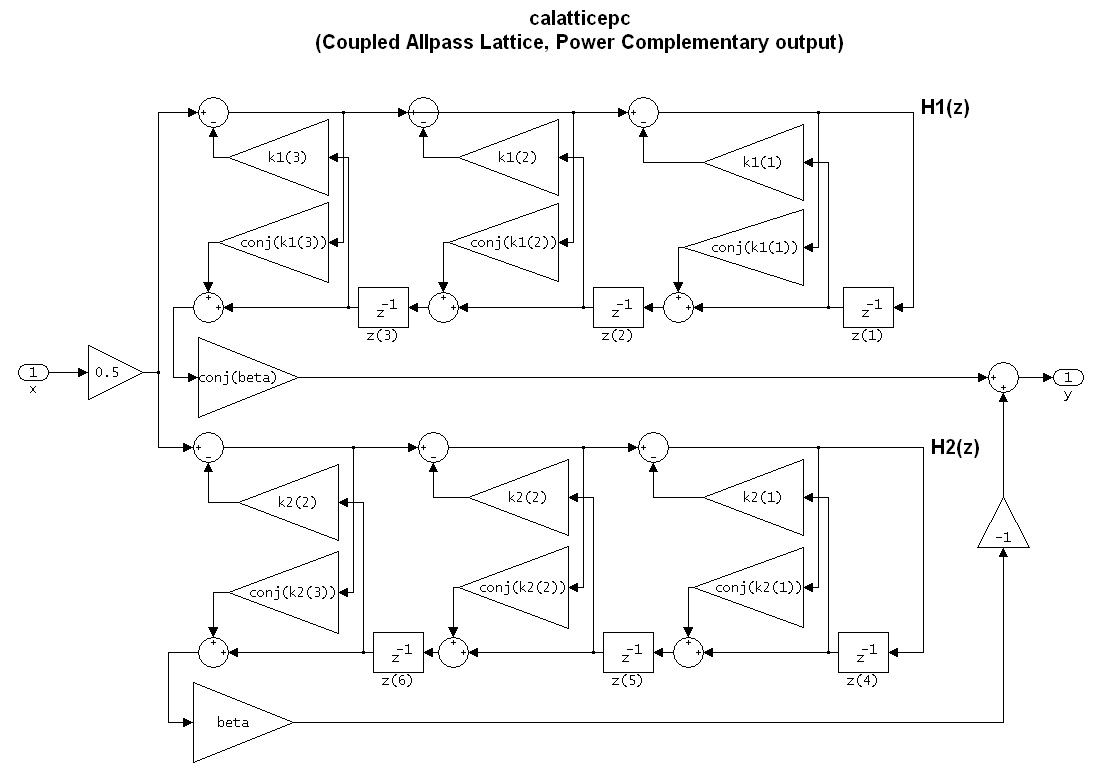Documentation

# dfilt.calatticepc

Coupled-allpass, power-complementary lattice filter

## Syntax

```hd = dfilt.calatticepc(k1,k2) hd = dfilt.calatticepc ```

## Description

`hd = dfilt.calatticepc(k1,k2)` returns a discrete-time, coupled-allpass, lattice filter object `hd`, with power-complementary output. This object is two allpass lattice filter structures coupled together to produce complementary output. The lattice coefficients for each structure are vectors, `k1` and `k2`, respectively. `beta` is shown in the following diagram.

`hd = dfilt.calatticepc` returns a default, discrete-time, coupled-allpass, lattice filter object `hd`, with power-complementary output. The default values are `k1` = `k2` = [], which is the default value for the `dfilt.latticeallpass`. The default for `beta` = 1. This filter passes the input through to the output unchanged.## Examples

Specify a third-order lattice coupled-allpass power complementary filter structure for a filter `hd` with the following code. You see from the returned properties that `Allpass1` and `Allpass2` contain vectors of coefficients for the constituent filters.

```k1 = [0.9511 + 0.3088i; 0.7511 + 0.1158i]; k2 = 0.7502 - 0.1218i; beta = 0.1385 + 0.9904i; hd = dfilt.calatticepc(k1,k2,beta); ```

To see the coefficients for `Allpass1`, check the property values.

```get(hd,'Allpass1') ```

Watch now